# Equation - math word problems

#### Number of problems found: 1351

• Square sides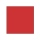If the square side increases by 28%, the square circumference increases by 11.2 meters. Find the length of the original square side.
• The truck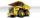The empty truck left the warehouse for goods at 10:00 and arrived at 11:30. At 2:00 pm, they loaded back on the same route. But it was a quarter slower than going to the warehouse. We know that on the way to the warehouse, the car was traveling at a speed
• Average temperatureThe average temperature from Monday to Sunday was 40.5°F and the average temperature from Monday to Saturday was 42.8°F. What was the temperature on Sunday?
• Every day 7 pagesAdelka reads the book every day 7 pages. When she reads one more page a day she will read it three days earlier. How long will Adelka read a book? How much does a book of pages have?
• Tickets1260 tickets sold. On the first day, 80% was sold on the second day was sold. How many tickets were sold first and how much the next day?
• There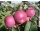There are 32 trees of three species in the orchard. 25% cherries, three eighty pears, others plums. How many plums are in the orchard?
• Ratio of two unknown numbersTwo numbers are given. Their sum is 30. We calculate one-sixth of a larger number and add to both numbers. So we get new numbers whose ratio is 5:7. Which two numbers were given?
• 12 apples12 apples and 2 loaves of bread cost 5.76 and 6 apples and 3 loaves of bread cost 7.68. How much is a loaf of bread?
• In and out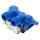The empty tank is filled in 12 minutes and empty in 16 minutes. How long does it take to fill if we forgot to close the drain? If the tank has 1000 l.
• Blueberries collecting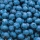Marie collects blueberries 2 l in 1 hour. Began collecting at 8.00 pm, Joe collects 1.5 l in 1 hour. Peter 1 l for 1 hr. And they began to gather at 08.30 pm. Together they collect 10 l. At what time will they all have collected?
• Mini-surveyIn the mini-survey of our class about the popularity of individual subjects, it turned out that 11.1% of pupils like mathematics, 18.5% are enjoying languages, 30.4% of pupils like physical education, and the remaining 12 pupils have several popular subje
• ParallelogramSide a of parallelogram is twice longer than side b. Its circumference is 78 dm. Calculate the length of the sides of a parallelogram.
• Ratio of sidesThe triangle has a circumference of 21 cm and the length of its sides is in a ratio of 6: 5: 3. Find the length of the longest side of the triangle in cm.
• The tubIf we drop the 5 l from the first tap and the from second 2 liters of water, the water in the tub will have a temperature of 25°C. If we drop 3 l from the first tap and from second 4 l of water, the water in the tub will have a temperature of 21°C. Determ
• Inlet minus outlet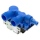Water flows into the tank through two pipes and flows out of the third pipe spontaneously. The pipe A itself would fill the tank in 3 hours, the pipe B itself would fille in 4 hours, and the pipe C itself would flow out in 12 hours. How long will the tank
• Father and sonFather and son weigh together 108kg. The father weighs 2.6 times more than his son. How much does weight my father and son count?
• ChildrenThe group has 42 children. There are 4 more boys than girls. How many boys and girls are in the group?
• SavingsEva borrowed 1/3 of her savings to her brother, 1/2 of savings spent in the store and 7 euros left. How much did she save?
• Class alphabet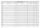All 29 pupils in the class are written in a class by alphabet. The number of pupils enrolled before Paul is three times higher than the number of pupils behind him. Calculate how many pupils are enrolled after Paul.
• Tank 11When 150 litres has been drawn from a tank it is 3/8 full, how many litres will the tank hold?

Do you have an interesting mathematical word problem that you can't solve it? Submit a math problem, and we can try to solve it.

We will send a solution to your e-mail address. Solved examples are also published here. Please enter the e-mail correctly and check whether you don't have a full mailbox.

Please do not submit problems from current active competitions such as Mathematical Olympiad, correspondence seminars etc...

Do you have a linear equation or system of equations and looking for its solution? Or do you have quadratic equation?